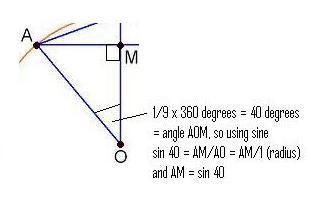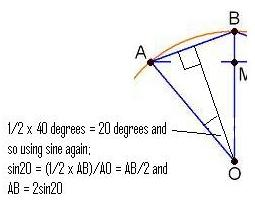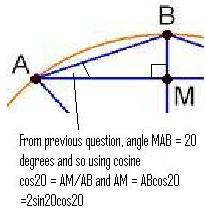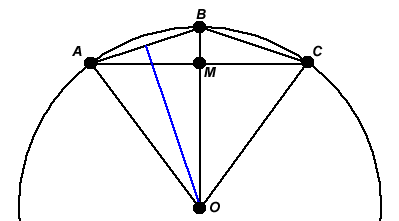#### You may also like### Coke Machine

The coke machine in college takes 50 pence pieces. It also takes a certain foreign coin of traditional design...### At a Glance

The area of a regular pentagon looks about twice as a big as the pentangle star drawn within it. Is it?### Cosines Rule

Three points A, B and C lie in this order on a line, and P is any point in the plane. Use the Cosine Rule to prove the following statement.

# Sine and Cosine for Connected Angles

##### Age 14 to 16Challenge Level

Chris from Saint John Payne School sent in clear diagrams to explain the first part.Diana thought about a general result. Here's what she sent us.In general, suppose that we've placed points $A$, $B$ and $C$ in such a way that $\angle A O B=\angle B O C=2\theta$. I'm going to show that $\sin(2\theta)=2\sin\theta\cos\theta$. This is called a double angle formula.\par From triangle $O A M$, we know that $A M=\sin(2\theta)$ (as the circle has radius $1$).

From triangle $O A B$, we know that $A B=2\sin\theta$ (the blue line bisects the angle at $O$ and since triangle $A O B$ is isosceles, the blue line meets $A B$ at a right angle, so we can think about two right-angled triangles, each with angle $\theta$ at $O$).

Since $\angle A B O=90^{\circ}-\theta$ (from the isosceles triangle $A O B$), we know that $\angle B A M=\theta$, and so from triangle $A B M$ we see that $A M=A B\cos\theta$.

Putting together the last two paragraphs, we get $A M=2\sin\theta\cos\theta$. But also $A M=\sin(2\theta)$, so $\sin(2\theta)=2\sin\theta\cos\theta$.